## Solve a Constrained Nonlinear Problem, Solver-Based

### Typical Optimization Problem

This example shows how to solve a constrained nonlinear problem using an Optimization Toolbox™ solver. The example demonstrates the typical workflow: create an objective function, create constraints, solve the problem, and examine the results.

This example provides two approaches to solving the problem. One uses the Optimize Live Editor task, a visual approach. The other uses the MATLAB® command line, a text-based approach. You can also solve this type of problem using the problem-based approach; see Solve a Constrained Nonlinear Problem, Problem-Based.

### Problem Formulation: Rosenbrock's Function

The problem is to minimize Rosenbrock's function

`$f\left(x\right)=100{\left({x}_{2}-{x}_{1}^{2}\right)}^{2}+{\left(1-{x}_{1}\right)}^{2},$`

over the unit disk, that is, the disk of radius 1 centered at the origin. In other words, find x that minimizes the function f(x) over the set ${x}_{1}^{2}+{x}_{2}^{2}\le 1$. This problem is a minimization of a nonlinear function with a nonlinear constraint.

Note

Rosenbrock's function is a standard test function in optimization. It has a unique minimum value of 0 attained at the point `[1,1]`. Finding the minimum is a challenge for some algorithms because the function has a shallow minimum inside a deeply curved valley. The solution for this problem is not at the point `[1,1]` because that point does not satisfy the constraint.

This figure shows two views of Rosenbrock's function in the unit disk. The vertical axis is log-scaled; in other words, the plot shows log(1+f(x)). Contour lines lie beneath the surface plot.

Rosenbrock's Function, Log-Scaled: Two Views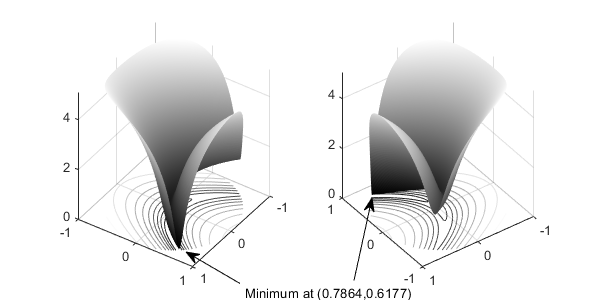The function f(x) is called the objective function. The objective function is the function you want to minimize. The inequality ${x}_{1}^{2}+{x}_{2}^{2}\le 1$ is called a constraint. Constraints limit the set of x over which a solver searches for a minimum. You can have any number of constraints, which are inequalities or equalities.

All Optimization Toolbox optimization functions minimize an objective function. To maximize a function f, apply an optimization routine to minimize –f. For more details about maximizing, see Maximizing an Objective.

### Define and Solve Problem Using Optimize Live Editor Task

The Optimize Live Editor task lets you set up and solve the problem using a visual approach.

1. Create a new live script by clicking the New Live Script button on the File section of the Home tab.2. Insert an Optimize Live Editor task. Click the Insert tab and then, in the Code section, select Task > Optimize.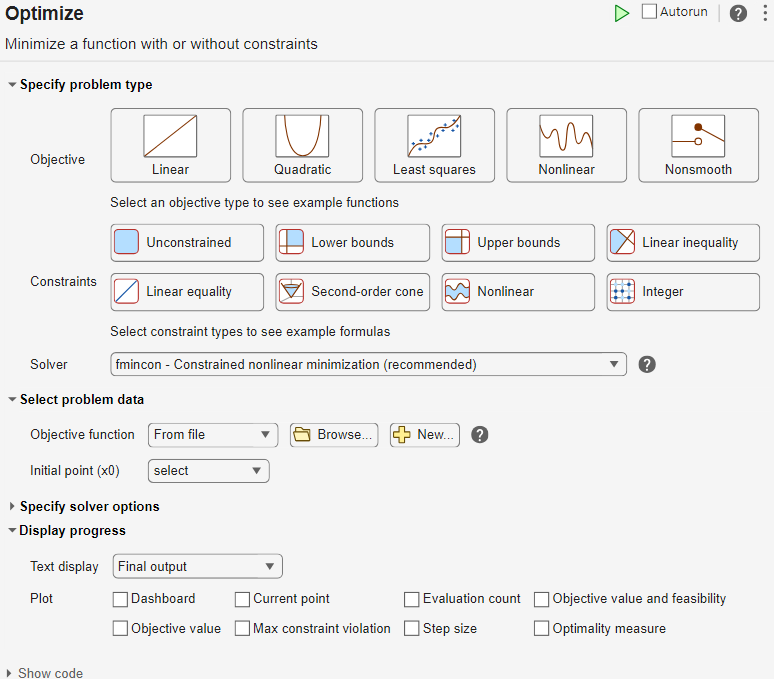3. In the Specify problem type section of the task, select Objective > Nonlinear and Constraints > Nonlinear. The task selects the solver ```fmincon - Constrained nonlinear minimization```.

4. Include Rosenbrock's function as the objective function. In the Select problem data section of the task, select Objective function > Local function and then click the button. A new local function appears in a section below the task.

```function f = objectiveFcn(optimInput) % Example: % Minimize Rosenbrock's function % f = 100*(y - x^2)^2 + (1 - x)^2 % Edit the lines below with your calculation x = optimInput(1); y = optimInput(2); f = 100*(y - x^2)^2 + (1 - x)^2; end```

This function implements Rosenbrock's function.

5. In the Select problem data section of the task, select Objective function > objectiveFcn.

6. Place the initial point `x0 = [0;0]` into the MATLAB workspace. Insert a new section above the Optimize task by clicking the task, then clicking the button on the Insert tab. In the new section above the task, enter the following code for the initial point.

`x0 = [0;0];`
7. Run the section by pressing Ctrl+Enter. This action places `x0` into the workspace.

8. In the Select problem data section of the task, select Initial point (x0) > x0.9. In the Select problem data section, select Constraints > Nonlinear > Local function and then click the button. A new local function appears below the previous local function.

10. Edit the new local function as follows.

```function [c,ceq] = unitdisk(x) c = x(1)^2 + x(2)^2 - 1; ceq = [ ]; end```
11. In the Select problem data section, select `unitdisk` as the constraint function.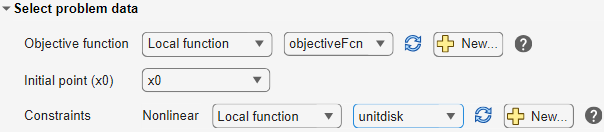12. To monitor the solver progress, in the Display progress section of the task, select Text display > Each iteration. Also, select for the plot.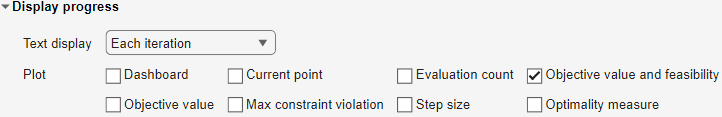13. To run the solver, click the options button at the top right of the task window, and select Run Section. The plot appears in a separate figure window and in the output area.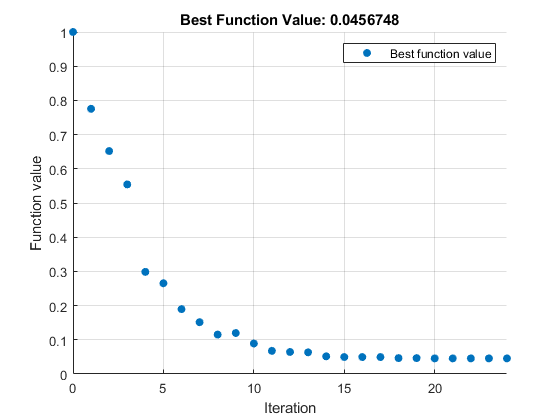The output area shows a table of iterations, discussed in Interpret Result.

14. To find the solution, look at the top of the task.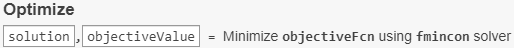The solver places the variables `solution` and `objectiveValue` in the workspace. View their values by inserting a new section break below the task and entering these lines.15. Run the section by pressing Ctrl+Enter.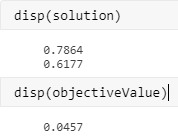To understand the `fmincon` process for obtaining the result, see Interpret Result.

16. To display the code that Optimize generates to solve the problem, click the options button at the top right of the task window, and select Controls and Code.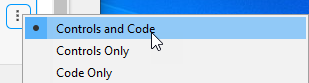At the bottom of the task, the following code appears.

```% Set nondefault solver options options = optimoptions('fmincon','Display','iter','PlotFcn',... 'optimplotfvalconstr'); % Solve [solution,objectiveValue] = fmincon(@objectiveFcn,x0,[],[],[],[],[],[],... @unitdisk,options);```

This code is the code you use to solve the problem at the command line, as described next.

### Define and Solve Problem at Command Line

The first step in solving an optimization problem at the command line is to choose a solver. Consult the Optimization Decision Table. For a problem with a nonlinear objective function and a nonlinear constraint, generally you use the `fmincon` solver.

Consult the `fmincon` function reference page. The solver syntax is as follows.

`[x,fval] = fmincon(fun,x0,A,b,Aeq,beq,lb,ub,nonlcon,options)`

The `fun` and `nonlcon` inputs represent the objective function and nonlinear constraint functions, respectively.

1. Define the objective function in the MATLAB language, as a function file or anonymous function. This example uses a function file.

2. Define the constraints as a separate file or anonymous function.

A function file is a text file that contains MATLAB commands and has the extension `.m`. Create a function file in any text editor, or use the built-in MATLAB Editor as in this example.

1. At the command line, enter:

`edit rosenbrock`
2. In the MATLAB Editor, enter:

```%% ROSENBROCK(x) expects a two-column matrix and returns a column vector % The output is the Rosenbrock function, which has a minimum at % (1,1) of value 0, and is strictly positive everywhere else. function f = rosenbrock(x) f = 100*(x(:,2) - x(:,1).^2).^2 + (1 - x(:,1)).^2;```

Note

`rosenbrock` is a vectorized function that can compute values for several points at once. See Vectorization. A vectorized function is best for plotting. For a nonvectorized version, enter:

```%% ROSENBROCK1(x) expects a two-element vector and returns a scalar % The output is the Rosenbrock function, which has a minimum at % (1,1) of value 0, and is strictly positive everywhere else. function f = rosenbrock1(x) f = 100*(x(2) - x(1)^2)^2 + (1 - x(1))^2;```
3. Save the file with the name `rosenbrock.m`.

Constraint functions have the form c(x) ≤ 0 or ceq(x) = 0. The constraint ${x}_{1}^{2}+{x}_{2}^{2}\le 1$ is not in the form that the solver handles. To have the correct syntax, reformulate the constraint as ${x}_{1}^{2}+{x}_{2}^{2}-1\le 0$.

The syntax for nonlinear constraints returns both equality and inequality constraints. This example includes only an inequality constraint, so you must pass an empty array `[]` as the equality constraint function ceq.

With these considerations in mind, write a function file for the nonlinear constraint.

1. Create a file named `unitdisk.m` containing the following code:

```function [c,ceq] = unitdisk(x) c = x(1)^2 + x(2)^2 - 1; ceq = [ ];```
2. Save the file `unitdisk.m`.

Now that you have defined the objective and constraint functions, create the other `fmincon` inputs.

1. Create options for `fmincon` to use the `'optimplotfvalconstr'` plot function and to return iterative display.

```options = optimoptions('fmincon',... 'PlotFcn','optimplotfvalconstr',... 'Display','iter');```
2. Create the initial point.

`x0 = [0 0];`
3. Create empty entries for the constraints that this example does not use.

```A = []; b = []; Aeq = []; beq = []; lb = []; ub = [];```

Solve the problem by calling `fmincon`.

`[x,fval] = fmincon(fun,x0,A,b,Aeq,beq,lb,ub,nonlcon,options)`
``` First-order Norm of Iter F-count f(x) Feasibility optimality step 0 3 1.000000e+00 0.000e+00 2.000e+00 1 13 7.753537e-01 0.000e+00 6.250e+00 1.768e-01 2 18 6.519648e-01 0.000e+00 9.048e+00 1.679e-01 3 21 5.543209e-01 0.000e+00 8.033e+00 1.203e-01 4 24 2.985207e-01 0.000e+00 1.790e+00 9.328e-02 5 27 2.653799e-01 0.000e+00 2.788e+00 5.723e-02 6 30 1.897216e-01 0.000e+00 2.311e+00 1.147e-01 7 33 1.513701e-01 0.000e+00 9.706e-01 5.764e-02 8 36 1.153330e-01 0.000e+00 1.127e+00 8.169e-02 9 39 1.198058e-01 0.000e+00 1.000e-01 1.522e-02 10 42 8.910052e-02 0.000e+00 8.378e-01 8.301e-02 11 45 6.771960e-02 0.000e+00 1.365e+00 7.149e-02 12 48 6.437664e-02 0.000e+00 1.146e-01 5.701e-03 13 51 6.329037e-02 0.000e+00 1.883e-02 3.774e-03 14 54 5.161934e-02 0.000e+00 3.016e-01 4.464e-02 15 57 4.964194e-02 0.000e+00 7.913e-02 7.894e-03 16 60 4.955404e-02 0.000e+00 5.462e-03 4.185e-04 17 63 4.954839e-02 0.000e+00 3.993e-03 2.208e-05 18 66 4.658289e-02 0.000e+00 1.318e-02 1.255e-02 19 69 4.647011e-02 0.000e+00 8.006e-04 4.940e-04 20 72 4.569141e-02 0.000e+00 3.136e-03 3.379e-03 21 75 4.568281e-02 0.000e+00 6.440e-05 3.974e-05 22 78 4.568281e-02 0.000e+00 8.000e-06 1.084e-07 23 81 4.567641e-02 0.000e+00 1.601e-06 2.793e-05 24 84 4.567482e-02 0.000e+00 2.023e-08 6.916e-06 Local minimum found that satisfies the constraints. Optimization completed because the objective function is non-decreasing in feasible directions, to within the value of the optimality tolerance, and constraints are satisfied to within the value of the constraint tolerance. x = 0.7864 0.6177 fval = 0.0457```The exit message tells you that the search for a constrained optimum ended because the derivative of the objective function is nearly 0 in directions allowed by the constraint, and that the constraint is satisfied to the required accuracy. Several phrases in the message contain links to more information about the terms used in the message. For more details about these links, see Enhanced Exit Messages.

### Interpret Result

The iteration table in both the Live Editor task output area and the MATLAB Command Window shows how MATLAB searched for the minimum value of Rosenbrock's function in the unit disk. Your table can differ, depending on toolbox version and computing platform. The following description applies to the table shown in this example.

• The first column, labeled `Iter`, is the iteration number from 0 to 24. `fmincon` took 24 iterations to converge.

• The second column, labeled `F-count`, reports the cumulative number of times Rosenbrock's function was evaluated. The final row shows an `F-count` of 84, indicating that `fmincon` evaluated Rosenbrock's function 84 times in the process of finding a minimum.

• The third column, labeled `f(x)`, displays the value of the objective function. The final value, `4.567482e-2`, is the minimum reported in the Optimize run, and at the end of the exit message in the Command Window.

• The fourth column, `Feasibility`, is 0 for all iterations. This column shows the value of the constraint function `unitdisk` at each iteration where the constraint is positive. Because the value of `unitdisk` was negative in all iterations, every iteration satisfied the constraint.

The other columns of the iteration table are described in Iterative Display.